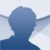# Gauthmath Android

1.5.0

## The Gauthmath application and its online tutors will help us to solve all types of mathematical problems with step-by-step explanations to get the answer

Can you imagine having a calculator that solves any kind of mathematical problem instantly, no matter how complicated it is? Well, that's exactly what the download of the APK file of this app offers us.

### Help for your maths homework in real time

Gauthmath is an educational tool that solves all kinds of mathematical problems and operations on the spot, and also offers a step-by-step solution. Therefore, it provides us with tailor-made assistance if we get stuck in a calculation or if we need help to do our maths homework.

Solve all mathematical problems in one application.

To use it, all you have to do is take a picture of the exercise or operation and indicate whether it is a problem or a calculation. Then, the automatic chat will open, scan the content of our image to digitise it and ask us if it is correct.

Once the data has been confirmed, the system will show the result within seconds. Not only that, but it also provides us with the steps to get to the answer and even graphs.

With real online tutors, you can get answers with step-by-step explanations to all your maths questions.

This maths problem solver helps us with trigonometry operations, functions, algebra, logic, matrices, calculus, geometry... but also with word problems. To do this, it has the help of online tutors available 24/7. Of course, we only have five free tickets, then we'll have to get our wallets out.